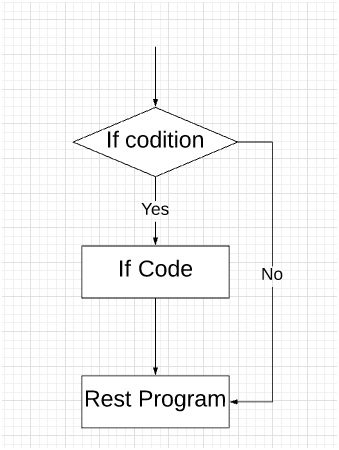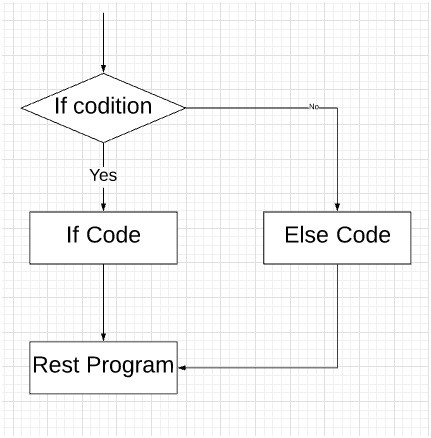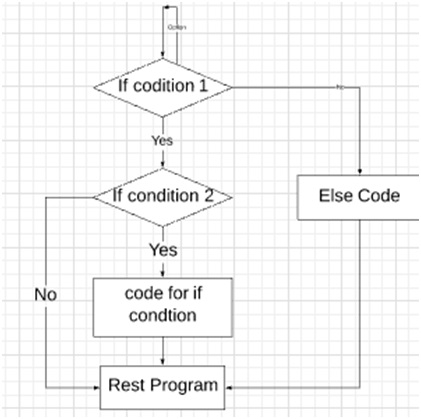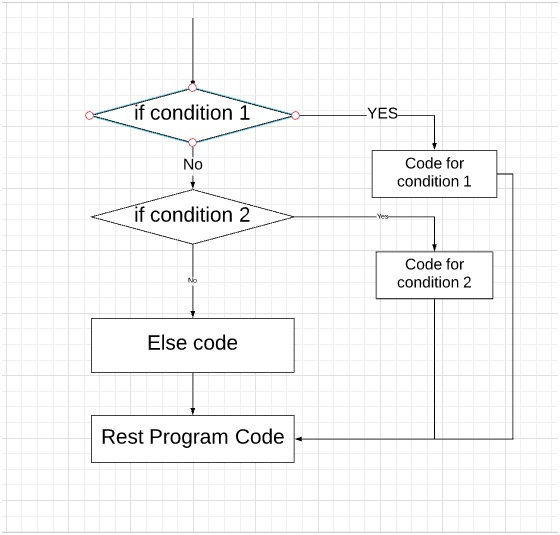# Conditional statements in Scala

Scala conditional statements: In this tutorial, we will learn about the various types of Scala conditional statements. Conditional statement execute only we a condition is true. We will see their use, types and example code.
Submitted by Shivang Yadav, on June 22, 2019

## Conditional statements in Scala

A conditional statement is the one which executes a block of code when a certain condition is fulfilled. Conditional statements help the program in decision making. Control statements or conditional statement decide which block of code need to be executed based on certain criteria.

In Scala, the following conditional statements are valid:

1. if condition: execute block if a condition is true.
2. if - else condition: execute a block if a condition is true otherwise execute another block.
3. Nested if-else: It checks for another condition inside the conditional block. If condition inside if condition.
4. if-else if Ladder: Used when multiple conditions need to be checked.

### 1) Scala if conditional statement

If the condition is used when the user needs to choose between only one condition. This lets program execute a block if the condition is true otherwise continue with the flow of the program.

Block execute if condition in the statement is TRUE otherwise nothing is done.

Syntax:

```    if(condition){
//Statement...
}
```

Control flow diagram:Example code:

```object MyClass {
def main(args: Array[String]) {
var myNumber = 21 ;
if(myNumber > 15){
print("Hey your number is bigger than fifteen")
}
}
}
```

Output

```Hey your number is bigger than fifteen
```

Example explanation:

In the above example, the If condition check weather myNumber is greater than 15. If it's greater than fifteen the code prints: "Hey your number is bigger than fifteen" If myNumber is not greater than 15 the code continues it general flow.

### 2) if-else conditional statement

if-else condition is used when the user needs to choose between two conditions. This lets program execute a block if the condition is true otherwise execute another block of code and then continue with the flow of the program.

Block execute if condition in the statement is TRUE otherwise another block of code is executed.

Syntax:

```    if(condition){
//Statement...
}
else{
//Statement
}
```

Control flow diagram:Example code:

```object MyClass {
def main(args: Array[String]) {
var myNumber = 21 ;
if(myNumber > 15){
print("Hey your number is bigger than fifteen")
}
else{
print("Hey your number is smaller than fifteen")
}
}
}
```

Output

```Hey your number is bigger than fifteen
```

Example explanation:

In the above example, the If condition check weather myNumber is greater than 15. If it's greater than fifteen the code prints: Hey your number is bigger than fifteen If myNumber is not greater than 15 the code prints: Hey your number is smaller than fifteen and then the code flow continues.

### 3) Nested if-else conditional statement

Nested if-else condition is used when the user needs to choose between a condition that is inside other condition. The flow goes into the first condition then inside the block of code anther condition which executes to execute another block of code.

Block execute if condition in the statement is TRUE otherwise another block of code is executed. If the first block of code is executed then inside this block there is another conditional statement that leads to another block of code.

Syntax:

```    if(condition 1){
if(condition 2){
//Statement
}
}
else{
//Statement
}
```

Control flow diagram:Example code:

```object MyClass {
def main(args: Array[String]) {
var myNumber = 21 ;
if(myNumber > 15){
print("Hey your number is bigger than fifteen")
}
}
else{
print("Hey your number is smaller than fifteen")
}
}
}
```

Output

```Hey your number is bigger than fifteen
```

Example explanation:

In this code, two variables are defined as myNumber and grade. First condition checks for myNumber, if it is greater than 15 than the flow gets into the block and prints "Hey your number is bigger than fifteen". then it checks the condition for the grade, if the grade is equal to "a" then it prints "Your grade is a". If myNumber is not greater than 15 then code execute the else statement that is Hey your number is smaller than fifteen. And finally goes through the rest code of the program.

In the if-else-if loop, the code flow into multiple conditions and if one goes FALSE, then another condition is executed and the condition checks go on. If all the conditions are FALSE, then finally, the else block is executed.

The flow goes on with checking conditions one by one. Until any one condition gets TRUE or the final else condition comes.

Syntax:

```    if(condition 1){
//Statement
}
else if(condition 2){
//Statement
}
else if(condition 3){
//Statement
}
.
.
.
else{
//Statement
}
```

Control flow diagram:Example code:

```object MyClass {
def main(args: Array[String]) {
var myNumber = 5 ;
if(myNumber > 20){
print("Hey your number is bigger than twenty")
}
else if(myNumber > 15){
print("Hey your number is bigger than fifteen")
}
else{
print("Hey your number is smaller than fifteen")
}
}
}
```

Output

```Hey your number is smaller than fifteen
```

Example explanation:

In this code, the program initializes one variable muNumber. Then it checks the value of myNumber to be greater than 20 in the first condition if the condition is true the code prints "Hey your number is bigger than Twenty". If this gets FALSE than it is checked for value to be greater than 15 in the second condition if this gets TRUE than the code prints "Hey your number is bigger than fifteen". At last, if all conditions are FALSE, it goes to the else condition that will execute when all are FALSE and the code prints "Hey your number is smaller than fifteen". After the execution of the else loop, the remaining code will get executed.

Languages: » C » C++ » C++ STL » Java » Data Structure » C#.Net » Android » Kotlin » SQL
Web Technologies: » PHP » Python » JavaScript » CSS » Ajax » Node.js » Web programming/HTML
Solved programs: » C » C++ » DS » Java » C#
Aptitude que. & ans.: » C » C++ » Java » DBMS
Interview que. & ans.: » C » Embedded C » Java » SEO » HR
CS Subjects: » CS Basics » O.S. » Networks » DBMS » Embedded Systems » Cloud Computing
» Machine learning » CS Organizations » Linux » DOS
More: » Articles » Puzzles » News/Updates